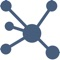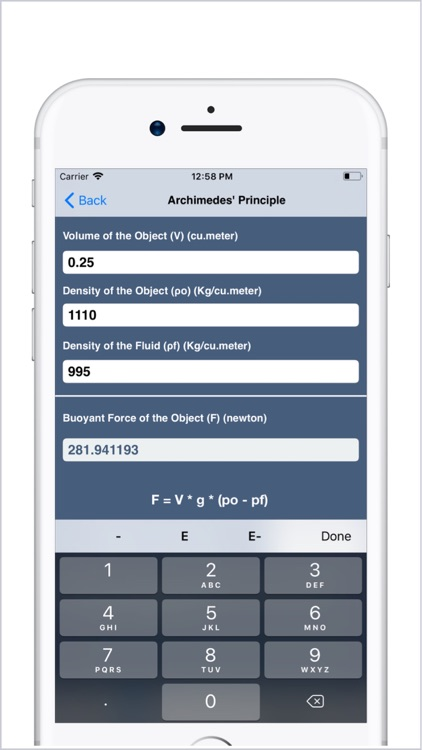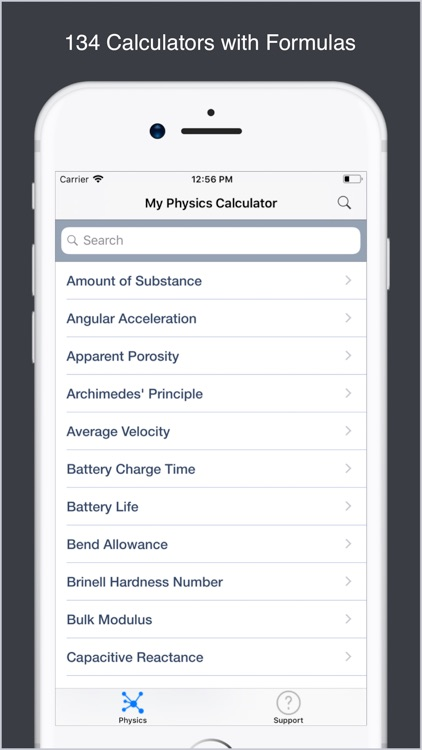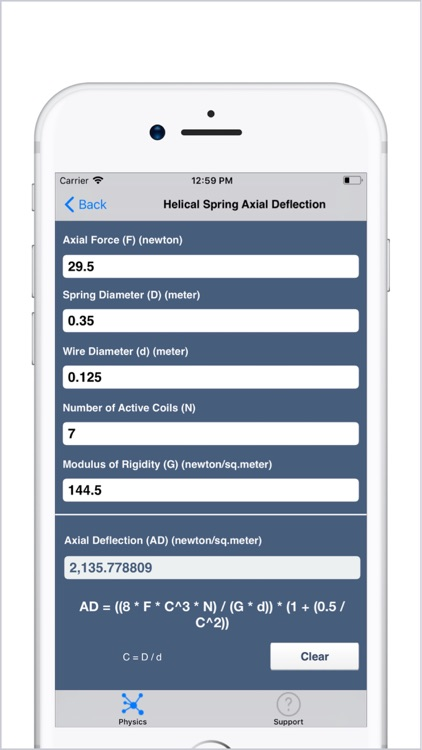## My Physics Calculator contains 134 Calculators, for different Physics and Engineering Parameters# My Physics Calculator

by V PUGAZHENTHIMy Physics Calculator contains 134 Calculators, for different Physics and Engineering Parameters. Simple User Interface with immediate results. Formulas and Definitions are provided for each calculator..### App Details

Version
6
Rating
(22)
Size
11Mb
Genre
Utilities Education
Last updated
November 18, 2018
Release date
March 5, 2014

### App Screenshots### App Store Description

My Physics Calculator contains 134 Calculators, for different Physics and Engineering Parameters. Simple User Interface with immediate results. Formulas and Definitions are provided for each calculator..
Most Comprehensive Physics & Engineering Calculator.

List of 134 Calculators:
• Force
• Kinetic Friction
• Static Friction
• Centripetal Force
• Centripetal Acceleration
• Gravitational Acceleration
• Angular Acceleration
• Work
• Total Work
• Power with Work
• Power with Displacement
• Power with Velocity
• Displacement or Distance
• Differential Pressure
• Density
• Water Density
• Kinetic Energy
• Potential Energy
• Elastic Potential Energy
• Einstein Mass Energy
• Gravitational Potential
• Velocity
• Circular Velocity
• Average Velocity
• Escape Velocity
• Drift Velocity
• Newton's Law of Gravity
• Newton's Second Law of Motion
• Archimedes' Principle
• Kepler's Third Law
• Hooke's Law
• Pascal's Law
• Poiseuille's Law
• Darcy's Law
• Stokes Law
• Souders-Brown Equation
• Podmore Factor
• Coulomb's Law
• Mirror Equation
• Cavitation Number
• Euler Number
• Fourier Number
• Knudsen Number
• Mach Number
• Nusselt Number
• Reynolds Number
• Weber Number
• Froude Number
• Prandtl Number
• Schmidt Number
• Brinell Hardness Number
• Doppler Effect - Wavelength Front
• Doppler Effect - Wavelength Behind
• Doppler Effect - Approaching Source
• Doppler Effect - Receding Source
• Doppler Effect - Approaching Receiver
• Doppler Effect - Receding Receiver
• Projectile Motion for Vertical Velocity
• Projectile Motion for Vertical Displacement
• Projectile Motion for Horizontal Displacement
• Projectile Motion for Range
• Impulse with Velocity
• Impulse with Time
• Momentum with Velocity
• Momentum with Time
• Moment
• Torque
• Moment of Inertia
• Transverse Strength
• Standard Surface Factor
• Rectangular Tank Capacity
• Cylinder Tank Capacity
• Apparent Porosity
• True porosity
• Kinematic Viscosity
• Mass Flow Rate
• Seismic Geophone
• Weight in Planets
• Wenner Spacing - Soil Resistivity
• Luminosity of Stars
• Temperature
• Thermal Conductivity
• Thermal Diffusivity
• Thermal Linear Expansion
• Thermal Volumetric Expansion
• Thermal Linear and Volumetric Relationship Expansion  • Heat Flow
• Heat Transfer Rate
• Specific Heat Capacity
• Sound Pressure Level
• Sound Intensity Level
• Sound Power Emitted
• Sound Wavelength
• Sound Speed
• RMS Noise
• Noise Pollution Level
• Simple Pendulum
• Physical Pendulum
• Leaf Springs
• Coincidence
• Helical Spring Rate
• Helical Spring Axial Deflection
• Helical Spring Index
• Amount of Substance
• Metric Weight
• Millspindle
• GSM of Paper
• D Exponent
• Bend Allowance
• Physics Constant Table
• Inductive Reactance
• Capacitive Reactance
• Resonant Frequency
• Inductor Sizing Equation
• Capacitor Sizing Equation
• Resistance
• Battery Life
• Battery Charge Time
• Kva
• Potentiometer
• Voltage Divider
• Electrodialysis
• Electrical Harmonics
• Horsepower
• Voltage (Ohm's Law)
• Power (Ohm's Law)
• Resistance (Ohm's Law)
• Current (Ohm's Law)
• Shear Modulus
• Bulk Modulus
• Youngs Modulus
• Stress
• Strain

Disclaimer:
AppAdvice does not own this application and only provides images and links contained in the iTunes Search API, to help our users find the best apps to download. If you are the developer of this app and would like your information removed, please send a request to [email protected] and your information will be removed.